# Taylor Series Using Horner’s Rule

## Taylor Series Using Horner’s Rule by Recursion in C Language

In this article, I am going to discuss Taylor Series using Horner’s Rule by recursion in C Language with Examples. Please read our previous article, where we discussed Taylor Series using recursion in C Language with Examples.

##### Understanding Taylor Series Using Horner’s Rule Concept:

In the previous article, we explained there Taylor Series by using the normal method of recursion. Here we will discuss Taylor Series by implementing Horner’s Rule in our recursive method. This method will be faster than the previous method of the Taylor Series. As this method requires less number multiplication then it takes less time to calculate the series. So, where the multiplication is done? Let’s have a look at the below equation.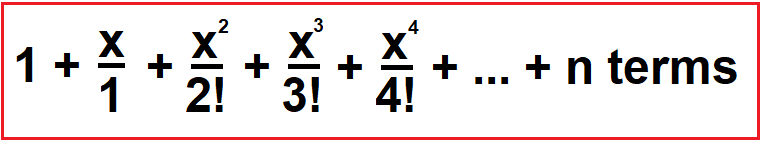In the above diagram, the multiplication takes place as: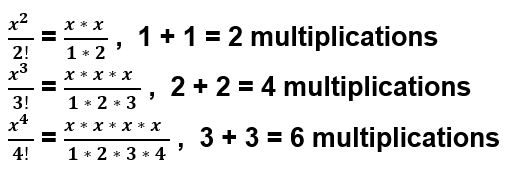In the above equations, multiplication occurs in power and factorial operations. We want to decrease the number of multiplications so that it takes less time to calculate the series with given parameters. As we observed the pattern of the number of multiplications in the above equations, Now, we can write an equation that shows how the number of multiplications increasing in the given series as:

2 + 4 + 6 + 8 + 10 ….

Now we take 2 commons from the above equation.

2 (1 + 2 + 3 + 4 + 5) …

Now the series is sum of first n natural number and we also know the formula for the same:

2 * n * (n + 1) / 2 = n (n + 1)

So, for calculating Taylor Series in the previous article, we require n (n + 1) number of multiplications and the Time Complexity is: O (n2).

We can say that n2 multiplication is required. Let’s jump to the formula which can reduce the number of multiplications in the Taylor Series.

##### Horner’s Rule:

The given equation is: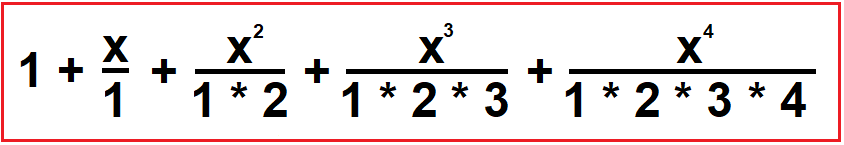Now, we take x/1 common from the series as:

###### Step 1: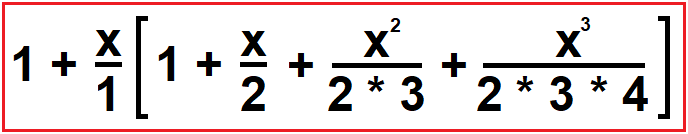Now, we take x/2 common from the series as:

###### Step 2: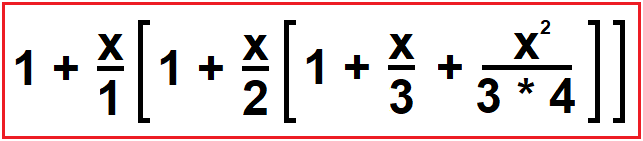The Time Complexity of the above equation is O (n2) i.e., Quadratic.

Now, we take x/3 common from the series as:

###### Step 3: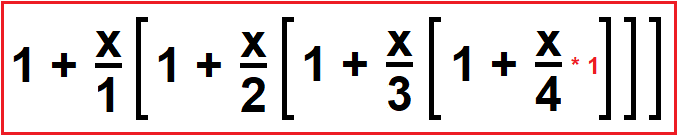The Time Complexity of the above equation is O (n) i.e., Linear.

Now, this is the required equation.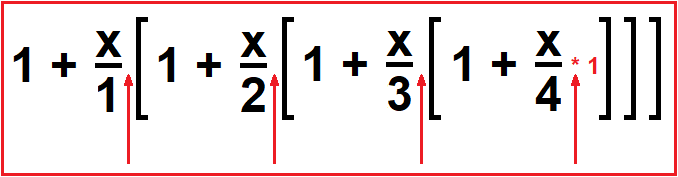Now as you can see here, we reduced the number of multiplications to 4 only (In the above diagram, the red arrow represents multiplication).

##### Example code:

Let’s have a look at the code.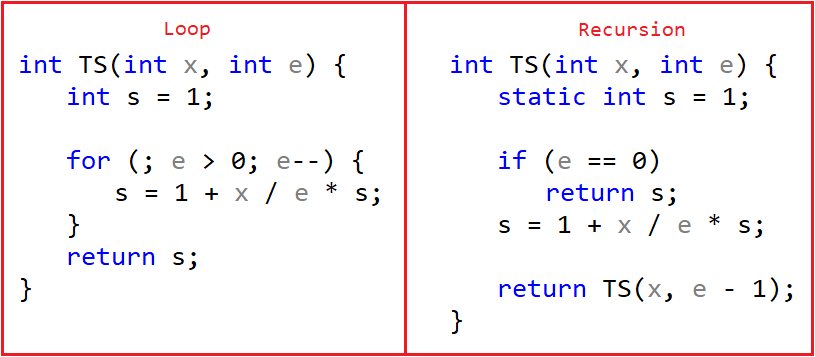There can be other ways also but we cover the most popular ways to implement Horner’s Rule. On the left-hand side of the above image, we used for loop and on the right-hand side, we simply use recursion here.

###### Taylor Series
```#include <stdio.h>
double e(int x, int n)
{
static double p = 1, f = 1;
double r;

if (n == 0)
return 1;
r = e (x, n - 1);
p = p * x;
f = f * n;
return r + p / f;
}

int main ()
{
printf ("%lf \n", e (4, 15));
return 0;
}
```
###### Taylor Series Horner’s Rule
```#include <stdio.h>
double e (int x, int n)
{
static double s;
if (n == 0)
return s;
s = 1 + x * s / n;
return e (x, n - 1);
}

int main ()
{
printf ("%lf \n", e (2, 10));
return 0;
}
```
###### Taylor Serie using Iterative
```#include <stdio.h>
double e (int x, int n)
{
double s = 1;
int i;
double num = 1;
double den = 1;

for (i = 1; i <= n; i++)
{
num *= x;
den *= i;
s += num / den;
}
return s;
}

int main ()
{
printf ("%lf \n", e (1, 10));
return 0;
}
```

In the next article, I am going to discuss the Combination Formula using Recursion in C Language with Examples. Here, in this article, I try to explain Taylor Series using Horner’s Rule in C Language with Example and I hope you enjoy this Taylor Series using Horner’s Rule in C Language with Example article.